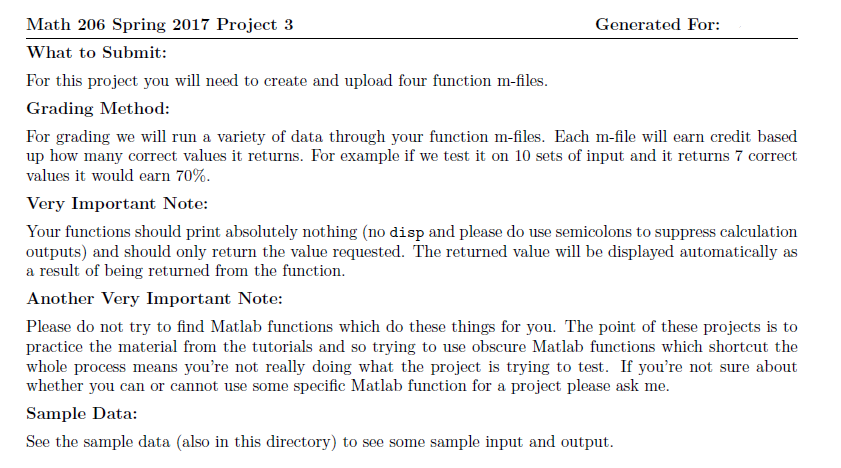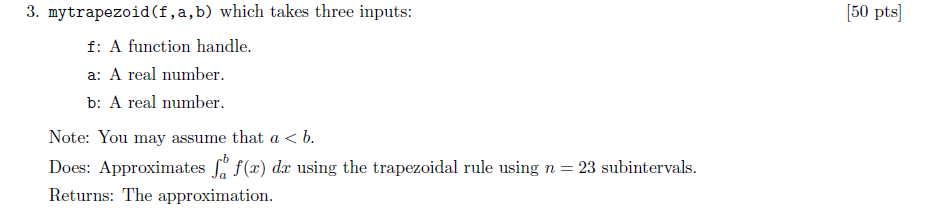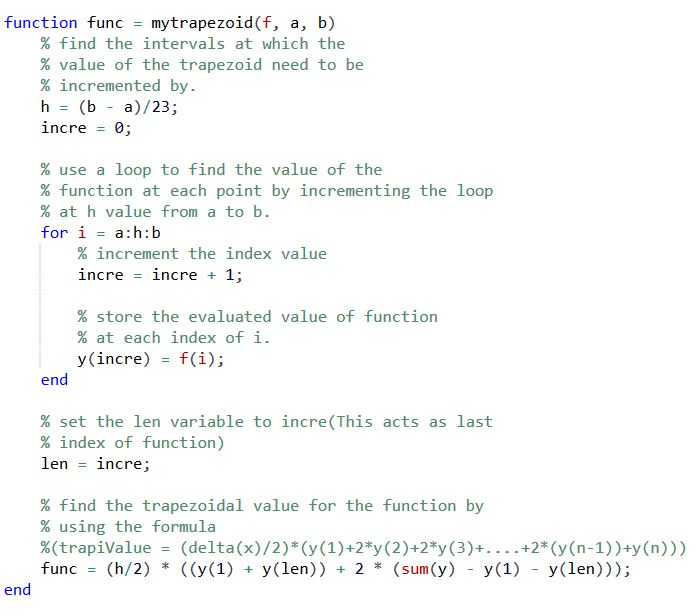# Question & Answer: THIS NEEDS TO BE…..

THIS NEEDS TO BE

ANSWERED IN MATLAB CODING ONLY please use screen shots so I can follow along because these two questions have been answered, but I do not understand how it works!

Don't use plagiarized sources. Get Your Custom Essay on
Question & Answer: THIS NEEDS TO BE…..
GET AN ESSAY WRITTEN FOR YOU FROM AS LOW AS \$13/PAGE

See problems under image below….

I KNOW HOW TO CALCULATE ALL OF THESE PROBLEMS ALREADY I DON’T KNOW HOW TO CODE IN MATLAB, so if possible show me the steps so I understand, Thank you.Generated For: Math 206 Spring 2017 Project 3 What to Submit: For this project you will need to create and upload four function m-files. Grading Method: For grading we will run a variety of data through your function m-fles. Each m-fle will earn credit based up how many correct values it returns. For example if we test it on 10 sets of input and it returns 7 correct values it would earn 70%. Very Important Note: Your functions should print absolutely nothing (no disp and please do use semicolons to suppress calculation outputs) and should only return the value requested. The returned value will be displayed automatically as a result of being returned from the function. Another Very Important Note: Please do not try to find Matlab functions which do these things for you. The point of these projects is to practice the material from the tutorials and so trying to use obscure Matlab functions which shortcut the whole process means you’re not really doing what the project is trying to test. If you’re not sure about whether you can or cannot use some specific Matlab function for a project please ask me. Sample Data: See the sample data (also in this directory) to see some sample input and output.

Screen shot of function trapezoidalValue.m:Here are the sample Runs for different function passing:

Sample Run – 1:

% define the function

f = @(x)1/(1+x);

% call the function by passing the function f, a = 0 and b = 10 values and display the resultant

mytrapezoid(f, 0, 10)

Sample output:

ans =  2.4132

Sample Run – 2:

% define the function

f = @(x) sin(2*x) + x/3;

% call the function by passing the function f, a = 0 and b = 10 values and display the resultant

mytrapezoid(f, 0, 10)

Sample Output:

ans =  16.944

Function trapezoidalValue.m code to copy:

function func = mytrapezoid(f, a, b)

% find the intervals at which the

% value of the trapezoid need to be

% incremented by.

h = (b – a)/23;

incre = 0;

% use a loop to find the value of the

% function at each point by incrementing the loop

% at h value from a to b.

for i = a:h:b

% increment the index value

incre = incre + 1;

% store the evaluated value of function

% at each index of i.

y(incre) = f(i);

end

% set the len variable to incre(This acts as last

% index of function)

len = incre;

% find the trapezoidal value for the function by

% using the formula

%(trapiValue = (delta(x)/2)*(y(1)+2*y(2)+2*y(3)+….+2*(y(n-1))+y(n)))

func = (h/2) * ((y(1) + y(len)) + 2 * (sum(y) – y(1) – y(len)));

end